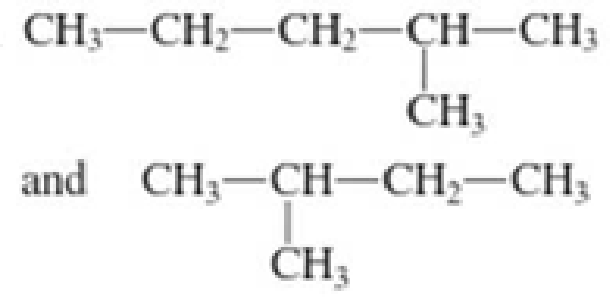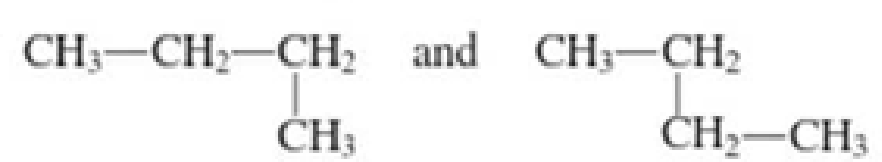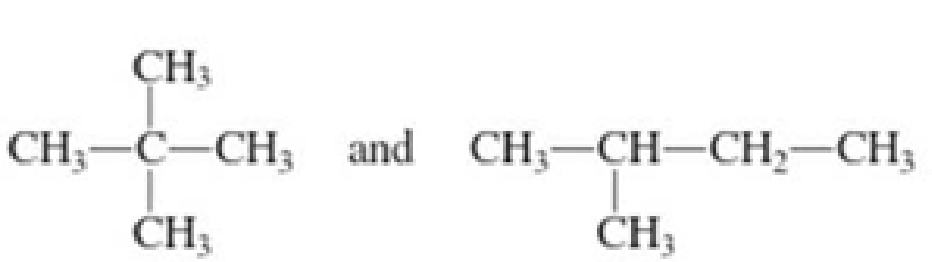Chapter 1, Problem 1.35EP### Organic And Biological Chemistry

7th Edition
STOKER + 1 other
ISBN: 9781305081079

#### Solutions

Chapter
Section### Organic And Biological Chemistry

7th Edition
STOKER + 1 other
ISBN: 9781305081079
Textbook Problem

# For each of the following pairs of structures, determine whether they are 1. Different conformations of the same molecule 2. Different compounds that are constitutional isomers 3. Different compounds that are not constitutional isomers a.b.c.d.(a)

Interpretation Introduction

Interpretation:

The given pair of structures are different conformations of same molecule or different compounds that are constitutional isomers or different compounds that are not constitutional isomers has to be determined.

Concept Introduction:

Organic compounds are represented shortly by the molecular formula and structural formula.  Each and every compound has its own molecular formula.  Compounds can have same molecular formula but not same structural formula.

Isomers are the compounds that have same molecular formula but different structural formula.  The main difference lies in the way the atoms are arranged in the structure.  Isomers have different chemical and physical properties even when they have same molecular formula.  This is known as Isomerism.

If there is difference only in the connectivity of the atoms in the molecule, then it is known as constitutional isomerism.  The isomers are known as constitutional isomers.  They will have same molecular formula and same functional group, but they differ in the connectivity between the atoms in the molecule.

Continuous-chain alkane is the one in which all the carbon atoms are bonded in a linear chain (non-branching chain).

Branched-chain alkane is an alkane which has atleast one branch in its structure that is attached to the continuous chain of carbon atoms.

When a single compound is represented in different orientations which is made possible by rotation about the single bonds in the molecule is known as conformations.

Explanation

The given structural formulas are,

Both the structural formulas contain different number of carbon atoms and hydrogen atoms.  Therefore, the molecular formulas of both the given structures are different.  The molecular formula for first structure is C

(b)

Interpretation Introduction

Interpretation:

The given pair of structures are different conformations of same molecule or different compounds that are constitutional isomers or different compounds that are not constitutional isomers has to be determined.

Concept Introduction:

Organic compounds are represented shortly by the molecular formula and structural formula.  Each and every compound has its own molecular formula.  Compounds can have same molecular formula but not same structural formula.

Isomers are the compounds that have same molecular formula but different structural formula.  The main difference lies in the way the atoms are arranged in the structure.  Isomers have different chemical and physical properties even when they have same molecular formula.  This is known as Isomerism.

If there is difference only in the connectivity of the atoms in the molecule, then it is known as constitutional isomerism.  The isomers are known as constitutional isomers.  They will have same molecular formula and same functional group, but they differ in the connectivity between the atoms in the molecule.

Continuous-chain alkane is the one in which all the carbon atoms are bonded in a linear chain (non-branching chain).

Branched-chain alkane is an alkane which has atleast one branch in its structure that is attached to the continuous chain of carbon atoms.

When a single compound is represented in different orientations which is made possible by rotation about the single bonds in the molecule is known as conformations.

(c)

Interpretation Introduction

Interpretation:

The given pair of structures is different conformations of same molecule or different compounds that are constitutional isomers or different compounds that are not constitutional isomers has to be determined.

Concept Introduction:

Organic compounds are represented shortly by the molecular formula and structural formula.  Each and every compound has its own molecular formula.  Compounds can have same molecular formula but not same structural formula.

Isomers are the compounds that have same molecular formula but different structural formula.  The main difference lies in the way the atoms are arranged in the structure.  Isomers have different chemical and physical properties even when they have same molecular formula.  This is known as Isomerism.

If there is difference only in the connectivity of the atoms in the molecule, then it is known as constitutional isomerism.  The isomers are known as constitutional isomers.  They will have same molecular formula and same functional group, but they differ in the connectivity between the atoms in the molecule.

Continuous-chain alkane is the one in which all the carbon atoms are bonded in a linear chain (non-branching chain).

Branched-chain alkane is an alkane which has atleast one branch in its structure that is attached to the continuous chain of carbon atoms.

When a single compound is represented in different orientations which is made possible by rotation about the single bonds in the molecule is known as conformations.

(d)

Interpretation Introduction

Interpretation:

The given pair of structures are different conformations of same molecule or different compounds that are constitutional isomers or different compounds that are not constitutional isomers has to be determined.

Concept Introduction:

Organic compounds are represented shortly by the molecular formula and structural formula.  Each and every compound has its own molecular formula.  Compounds can have same molecular formula but not same structural formula.

Isomers are the compounds that have same molecular formula but different structural formula.  The main difference lies in the way the atoms are arranged in the structure.  Isomers have different chemical and physical properties even when they have same molecular formula.  This is known as Isomerism.

If there is difference only in the connectivity of the atoms in the molecule, then it is known as constitutional isomerism.  The isomers are known as constitutional isomers.  They will have same molecular formula and same functional group, but they differ in the connectivity between the atoms in the molecule.

Continuous-chain alkane is the one in which all the carbon atoms are bonded in a linear chain (non-branching chain).

Branched-chain alkane is an alkane which has atleast one branch in its structure that is attached to the continuous chain of carbon atoms.

When a single compound is represented in different orientations which is made possible by rotation about the single bonds in the molecule is known as conformations.

### Still sussing out bartleby?

Check out a sample textbook solution.

See a sample solution

#### The Solution to Your Study Problems

Bartleby provides explanations to thousands of textbook problems written by our experts, many with advanced degrees!

Get Started